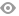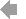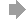﻿ Page 15 - MATINF Nr. 6
Basic HTML VersionView Full Version
Page 15 - MATINF Nr. 6
P. 15

Proof for AM-GM inequality using calculus tehniques

Dorin M˘arghidanu       1

There are many proofs for the well known inequality between arithmetic mean and geometric
mean, or Cauchy’s inequality. See  and the more extensive bibliography contained therein.
In this paper we shall give a new one. For this, we will give two preliminaries calculus results.

Although the ﬁrst result is known, we recall it - as a basis for the next.
Lemma 1. For x > 1, holds the following inequality, e    1−  1 x < x<e x−1 .
Proof. We consider the function f : [1, ∞) → [1, ∞), f(x) = ln x and apply Lagrange’s theorem
lnx    1
on the interval [1, x] ⊂ [1, ∞). There is c ∈ (1, x) so that     = .
x−1     c
1 1                1   lnx           x−1
Since   < <1, it comes     <     <1, thus       <ln x<x−1.
x c                x x−1               x
By exponentiation we get indeed the inequality stated earlier.
An inequality - interesting in itself - is stated in.

b−a  b    b−a
Lemma 2. For 0 < a ≤ b, occurs the following e      b ≤ ≤e a .
a
a
Proof. For a = b, we have obviously an equality. Supposing that b > a, then with x= >1 in
b
Lemma 1, it comes immediately the double inequality in Lemma 2.
∗
∗
Theorem 1. (Cauchy’s inequality or AM-GM inequality.) If x 1 , x 2 , . . . , x n ∈ R , n ∈ N and
+
√
x 1 + x 2 + . . . + x n
A :=                      , G :=   n  x 1 · x 2 · . . . · x n , then A ≥ G, with equality if and only if
n
x 1 = x 2 = . . . = x n .
∗
Proof. For h ∈ N , we note: P h := x 1 · x 2 · . . . · x h , S h := x 1 + x 2 + . . . + x h ; thus A =  S n  ,
√                                                                                       n
G :=   n  P n . Without restricting the generality, we suppose that 0 < x 1 ≤ x 2 ≤ . . . ≤ x n . Since
∗
min{x 1 , x 2 , . . . , x n } ≤ G ≤ max{x 1 , x 2 , . . . , x n } results that exist k ∈ N so that
0 < x 1 ≤ x 2 ≤ . . . ≤ x n ≤ G ≤ x k+1 ≤ . . . ≤ x n                (1)
By it’s very name – of (geometric) mean, G justiﬁes it’s intermediate position!). With (1) and
the left inequality in Lemma 2, we have:
G
G−x 1
x 1 ≤ G → e   G   ≤                                     (2 1 )
x 1
G
G−x 2
x 2 ≤ G → e   G   ≤                                     (2 2 )
x 2
. . .

G−x k   G
x k ≤ G → e   G   ≤                                     (2 k )
x k
1
Profesor dr., Colegiul Nat , ional ,,Al. I. Cuza”, Corabia, d.marghidanu@gmail.com

1510   11   12   13   14   15   16   17   18   19   20